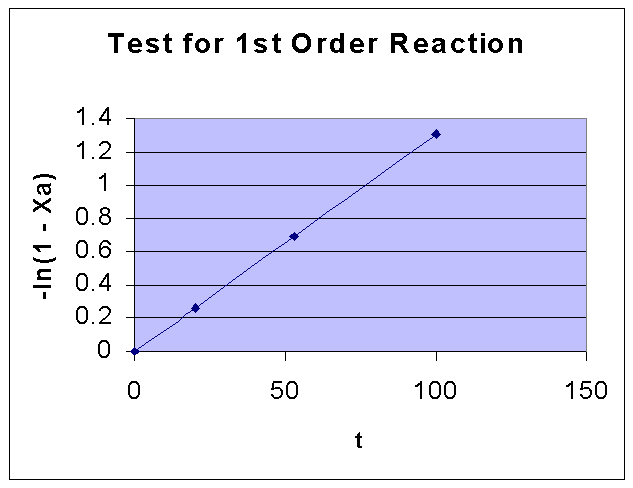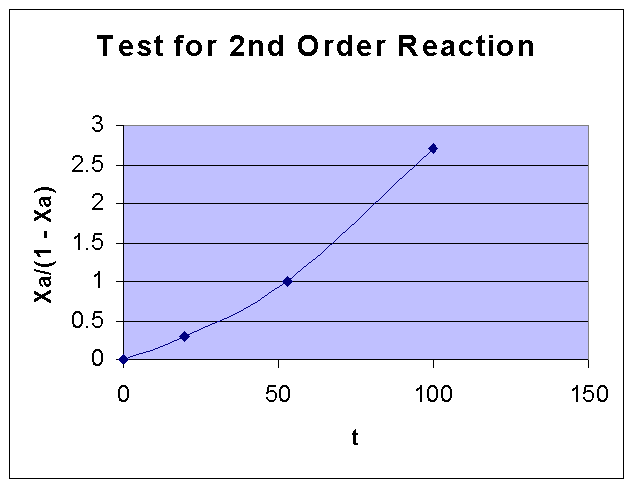### Kinetics of Thermal Decomposition of N2O

Establish the kinetics of the thermal decomposition of Nitrous oxide from the following data and find the reaction rate at 990oC and an initial pressure of 200 mm Hg.

```
t, min		20	53	100

% decomposition	23	50	73
```

Calculations:

Conversion (XA) of Nitrous oxide (A) for various time values are given are given.

We shall test the date for first order, second order kinetics by integral method of analysis.

For a first order reaction, -ln(1 - XA) vs. t is a straight line.

```t, min	20	53	100

XA	0.23	0.50	0.73```

 t, min 20 53 100 XA 0.23 0.5 0.73 1-XA 0.77 0.5 0.27 -ln(1 - XA) 0.261365 0.693147 1.30933

From the above data the following graph is drawn:For a second order reaction,

XA/(CAo (1- XA) ) = kt

i.e., XA/(1 - XA) vs. t is a straight line

 t, min 20 53 100 XA/(1 - XA) 0.298701 1 2.7037

From the above data the following graph is drawn:By comparing the two graphs, it can be seen that First order kinetics is well fiiting the given data. Fisrt order rate constant k is obtained from the slope of the first order data graph, and it is = 0.0131 sec-1.

Therefore, -rA = kCA = kCAo(1 - XA) = kpAo(1 - XA)/RT = (1 - XA) x 0.0131 x (200/760) x 101325 /(8314 x (990 + 273)) = 3.327 x 10-5(1 - XA)

i.e., -rA = 3.327 x 10-5(1 - XA)

where XA is fractional decomposition of Nitrous oxide, and -rA is the rate of decomposition of Nitrous oxide.